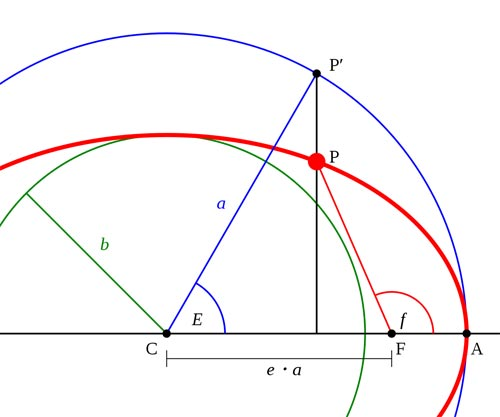A# anomalyFig 1. The true anomaly of point P is the angle f. The center of the ellipse is point C, and the focus is point F.

The anomaly is an angle that gives the position of an object in an elliptical orbit at any given time.

The true anomaly (Fig 1)is the angle between the periapsis of an orbit and the object's current orbital position, measured from the body being orbited and in the direction of orbital motion.

The mean anomaly is what the true anomaly would be if the object orbited in a perfect circle at constant speed. The mean anomaly is 0° at periapsis and 180° at apoapsis, just as for the true anomaly, but at other points along the orbit the values of the mean and true anomalies differ. The mean anomaly at a given time is often used as an orbital element.

The eccentric anomaly is an angle related to both the true anomaly and the mean anomaly that is encountered when solving Kepler's equation.